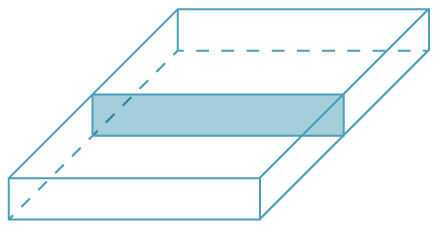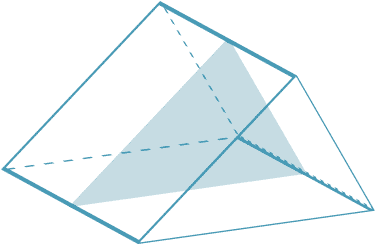## Prisms

A polyhedron is a solid bounded by polygons. A right prism is a polyhedron that has two congruent and parallel faces (called the base and top), with all its remaining faces rectangles. This means that when a right prism is stood on its base, all the walls are vertical rectangles. We generally say 'prism' when we really mean 'right prism'. A prism has uniform cross-section. This means that when you take slices through the solid parallel to the base, you get polygons congruent to the base. So the area of each slice is always the same.

In a rectangular prism, the cross-section is always a rectangle.With a triangular prism, the cross-section is always a triangle.